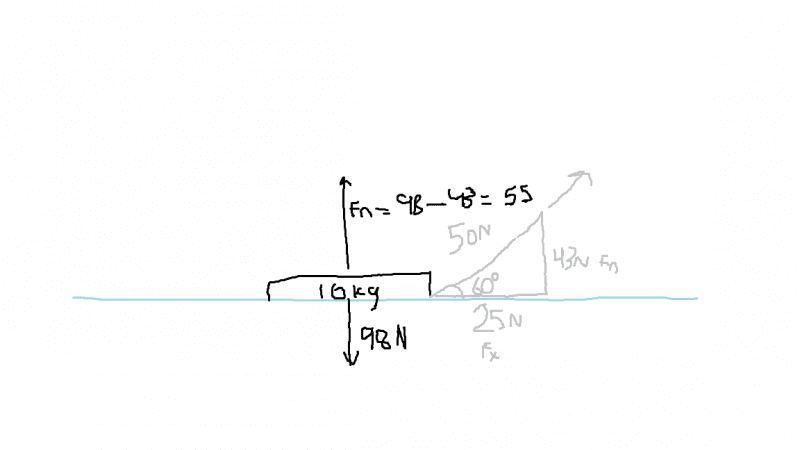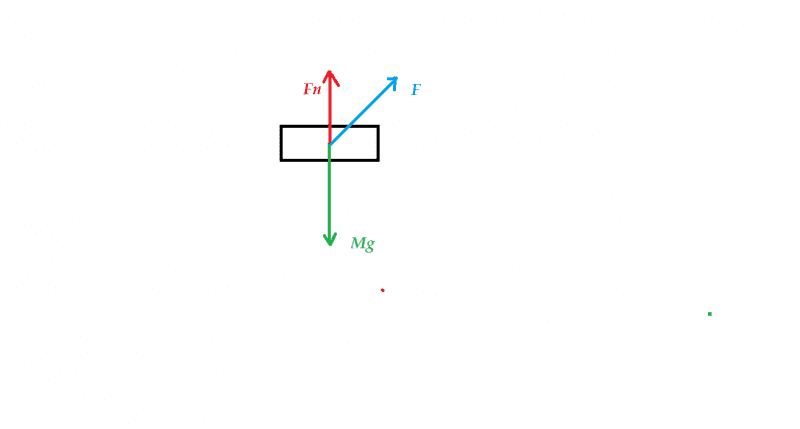# Find force of friction given mass, angular force, and undefined constant velocity

## Homework Statement

A child is pulling a 10kg sled along a horizontal plane of ice with a force of 50N directed 60 degrees north of east. Find the force of friction between the ice and the sled, given that the sled is moving at a constant velocity.

Fnet = ma
Fnet = Fx - Fk
muK = Fk/Fn

## The Attempt at a Solution

Here's kind of a crappy drawing of what I was able to get out of this...I just dont know where to go from here. I have Fx, 25N, and I believe Fn is 55N, but to find Fk i need to find Fnet, which is where I get stuck. And I don't know how knowing that the object is moving at a constant velocity can help me when I don't actually know it's magnitude. Any help is appreciated!

## Answers and Replies

Orodruin
Staff Emeritus
Science Advisor
Homework Helper
Gold Member
What does Newton's laws say about an object which is moving at a constant velocity?

It will stay at that velocity unless something acts upon it with net force?

What does Newton's laws say about an object which is moving at a constant velocity?
It will stay at that velocity unless something acts upon it with net force?

I think I found it: since the object is at constant velocity, the acceleration is 0, so Fnet= 10 (0) = 0
So Fk = 25 (Fnet=Fx-Fk) and then muK = 25/Fn. I realize that, in my drawing, I accidentally subtracted from Fn instead of adding to it, so Fn is 141.
muK = 25/141 = .18

haruspex
Science Advisor
Homework Helper
Gold Member
2020 Award
I think I found it: since the object is at constant velocity, the acceleration is 0, so Fnet= 10 (0) = 0
So Fk = 25 (Fnet=Fx-Fk) and then muK = 25/Fn. I realize that, in my drawing, I accidentally subtracted from Fn instead of adding to it, so Fn is 141.
muK = 25/141 = .18
If your drawing is correct then you were right to subtract the 43N from mg to obtain the normal force. The force of gravity on the sled is balanced by the combination of the upward normal force and the upward pull of the string.
However, the problem statement says the string is oriented 60 degrees north of east, not 60 degrees above the horizontal.

Orodruin
Staff Emeritus
Science Advisor
Homework Helper
Gold Member
Exactly. No acceleration (constant velocity) means no net force. However, note that (if you wrote down the problem exactly as stated) you could have stopped when you had found the force of friction. Although there is nothing wrong in doing more, be mindful of what you put in the answer. Otherwise, see haruspex' reply.

If your drawing is correct then you were right to subtract the 43N from mg to obtain the normal force. The force of gravity on the sled is balanced by the combination of the upward normal force and the upward pull of the string.
However, the problem statement says the string is oriented 60 degrees north of east, not 60 degrees above the horizontal.
Why do you subtract 43 from Fn instead of adding? Arent both forces moving in the same direction?

Why do you subtract 43 from Fn instead of adding? Arent both forces moving in the same direction?
He has asked you to subtract 43N from mg(98N).Are they in same direction?

He has asked you to subtract 43N from mg(98N).Are they in same direction?
Isn't Fn the equal but opposite force opposing the force of gravity, meaning it is directed upwards along with the upward force created by the rope?

Isn't Fn the equal but opposite force opposing the force of gravity, meaning it is directed upwards along with the upward force created by the rope?Fn is not equal to Mg.
The block's acceleration in vertical direction is 0.So net force in vertical direction should be 0.
Can you write an equation for this?